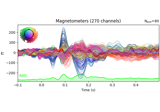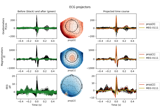# mne.compute_proj_epochs#

mne.compute_proj_epochs(epochs, n_grad=2, n_mag=2, n_eeg=2, n_jobs=None, desc_prefix=None, meg='separate', verbose=None)[source]#

Compute SSP (signal-space projection) vectors on epoched data.

This function aims to find those SSP vectors that will project out the `n` most prominent signals from the data for each specified sensor type. Consequently, if the provided input data contains high levels of noise, the produced SSP vectors can then be used to eliminate that noise from the data.

Parameters:
epochsinstance of `Epochs`

The epochs containing the artifact.

n_grad`int` | `float` between `0` and `1`

Number of vectors for gradiometers. Either an integer or a float between 0 and 1 to select the number of vectors to explain the cumulative variance greater than `n_grad`.

n_mag`int` | `float` between `0` and `1`

Number of vectors for magnetometers. Either an integer or a float between 0 and 1 to select the number of vectors to explain the cumulative variance greater than `n_mag`.

n_eeg`int` | `float` between `0` and `1`

Number of vectors for EEG channels. Either an integer or a float between 0 and 1 to select the number of vectors to explain the cumulative variance greater than `n_eeg`.

n_jobs

The number of jobs to run in parallel. If `-1`, it is set to the number of CPU cores. Requires the `joblib` package. `None` (default) is a marker for ‘unset’ that will be interpreted as `n_jobs=1` (sequential execution) unless the call is performed under a `joblib.parallel_config` context manager that sets another value for `n_jobs`. Number of jobs to use to compute covariance.

desc_prefix

The description prefix to use. If None, one will be created based on the event_id, tmin, and tmax.

meg`str`

Can be `'separate'` (default) or `'combined'` to compute projectors for magnetometers and gradiometers separately or jointly. If `'combined'`, `n_mag == n_grad` is required and the number of projectors computed for MEG will be `n_mag`.

New in v0.18.

verbose

Control verbosity of the logging output. If `None`, use the default verbosity level. See the logging documentation and `mne.verbose()` for details. Should only be passed as a keyword argument.

Returns:
projs: `list` of `Projection`

List of projection vectors.

## Examples using `mne.compute_proj_epochs`#Working with CTF data: the Brainstorm auditory dataset

Working with CTF data: the Brainstorm auditory datasetRepairing artifacts with SSP

Repairing artifacts with SSP